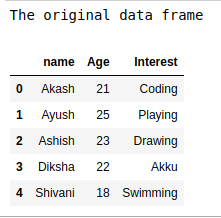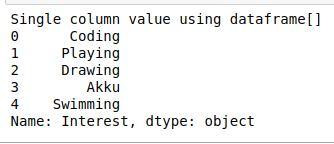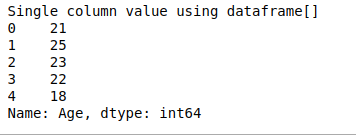# How to Select single column of a Pandas Dataframe?

In this article, we will discuss how to select a single column in a data frame. Now let us try to implement this using Python.

First, let’s create a dataframe

## Python3

 `# importing pandas as library ` `import` `pandas as pd ` ` `  ` `  `# creating data frame: ` `df ``=` `pd.DataFrame({``'name'``: [``'Akash'``, ``'Ayush'``, ``'Ashish'``, ` `                            ``'Diksha'``, ``'Shivani'``], ` `                    `  `                   ``'Age'``: [``21``, ``25``, ``23``, ``22``, ``18``], ` `                    `  `                   ``'Interest'``: [``'Coding'``, ``'Playing'``, ``'Drawing'``, ` `                                ``'Akku'``, ``'Swimming'``]}) ` ` `  `print``(``"The original data frame"``) ` `df`

Output:Method 1: Using Dot(dataframe.columnname) returns the complete selected column

## Python3

 `# using dot method ` `print``(``"Single column value using dataframe.dot"``) ` `print``(df.Interest) `

Output:Method 2: Using dataframe[columnname] method:
There are some problems that may occur with using dataframe.dot are as follows:

• Through dot method, we cannot Select column names with spaces.
• Ambiguity may occur when we Select column names that have the same name as methods for example max method of dataframe.
• We cannot Select multiple columns using dot method.
• We cannot Set new columns using dot method.

Because of the above reason dataframe[columnname] method is used widely.

## Python3

 `# using dataframe[columnname]method ` `print``(``"Single column value using dataframe[]"``) ` `print``(df[``'Interest'``]) `

Output:Another Example now if we want to select the column Age.

## Python3

 `# using dataframe[columnname]method ` `print``(``"Single column value using dataframe[]"``) ` `print``(df[``'Age'``]) `

Output:Whether you're preparing for your first job interview or aiming to upskill in this ever-evolving tech landscape, GeeksforGeeks Courses are your key to success. We provide top-quality content at affordable prices, all geared towards accelerating your growth in a time-bound manner. Join the millions we've already empowered, and we're here to do the same for you. Don't miss out - check it out now!